# 30 degree angle

30 degree angle
Go back to  'Angles'

In this mini-lesson, we will learn about 30-degree angle by understanding the rules to draw it using a protractor, its real-life examples, and how to apply them while solving problems. We will also discover interesting facts around them.

Have you ever noticed triangular shapes in your surroundings?

Did you know that if we divide the 60-degree angle into two parts, we have a 30-degree angle?

30-degree angles have a very significant role in real-life applications.

For example, there is a 30-degree rule in film making. Cameramen have to place the camera at 30 degrees with respect to the ground to capture more beautiful photos and videos.

In this mini-lesson, let us explore the 30-degree angle!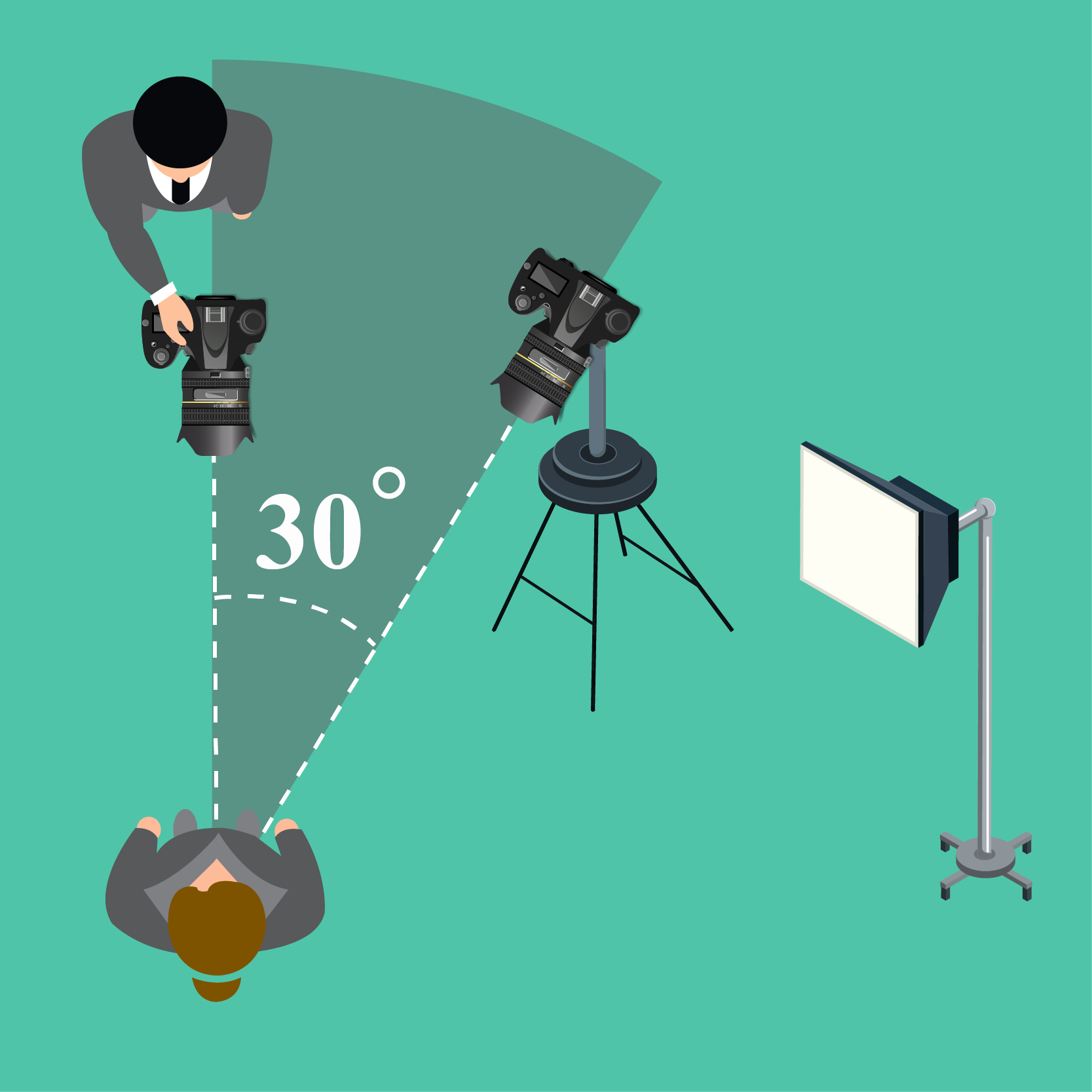## What Is a 30-Degree Angle?

An angle is formed when two rays meet at a point.

The point of intersection of the ray OA and ray OB is O, which is called the vertex.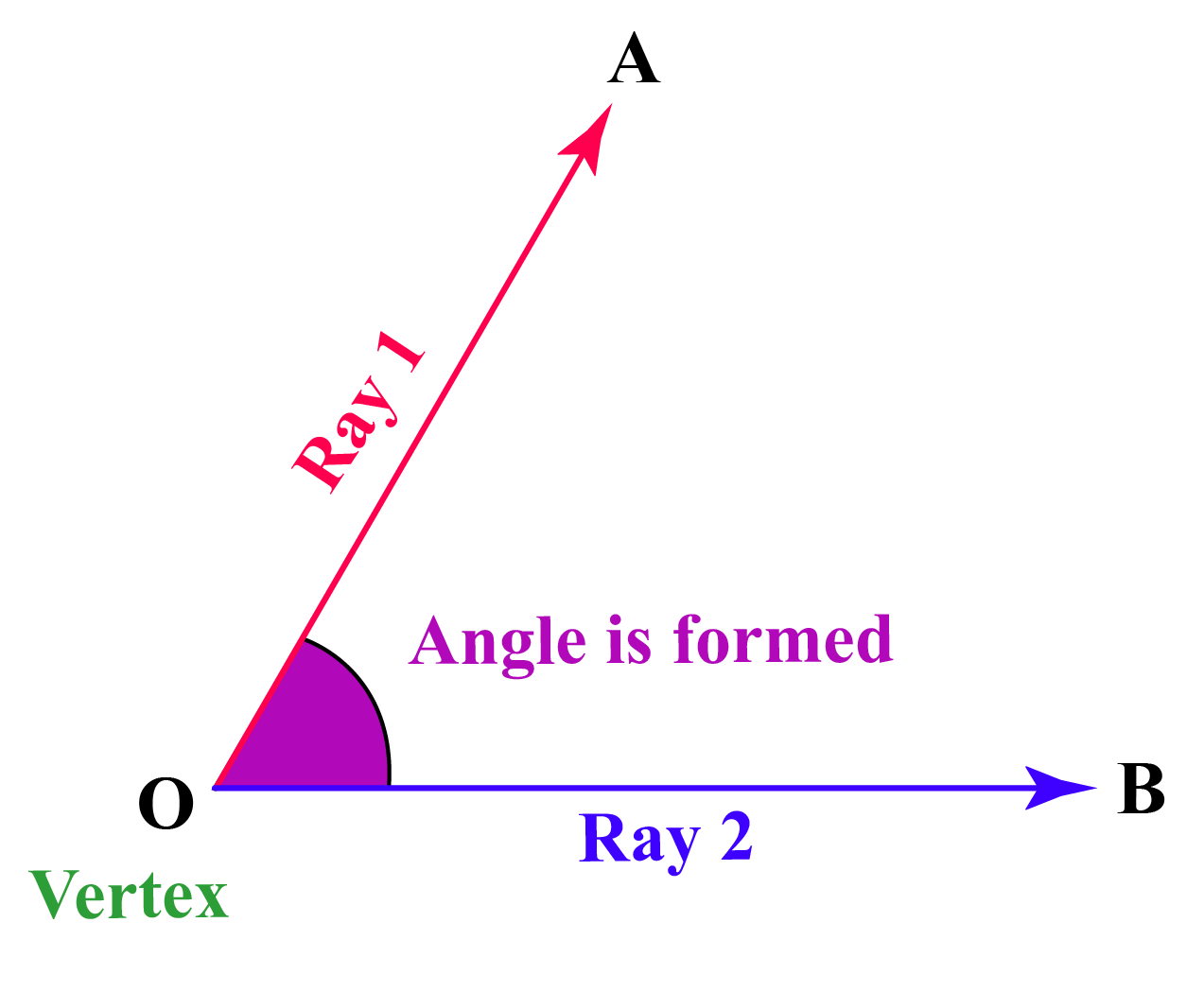If the measure of the angle formed by two rays is 30 degrees, then the angle is called a 30-degree angle.

The angle formed by the ray OA and OB is written as $$\angle \text{AOB}$$ or $$\angle \text{BOA}$$.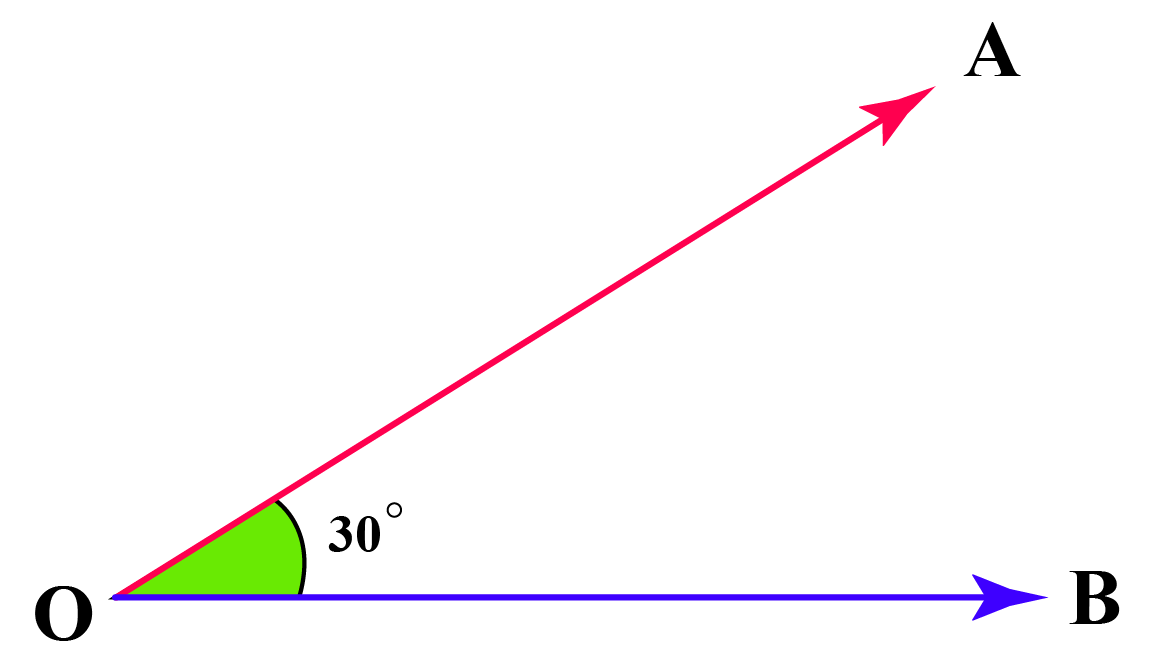$\angle \text{AOB} = \angle \text{BOA} = 30^{\circ}$

## How to Construct a 30-Degree Angle Using a Protractor?

In this section, let us explore how to construct a 30-degree angle with the help of a protractor.

Step 1: Draw a line segment $$OA$$.

Step 2: Place the center tip of the protractor at point $$O$$ such that the protractor perfectly aligns with line AO.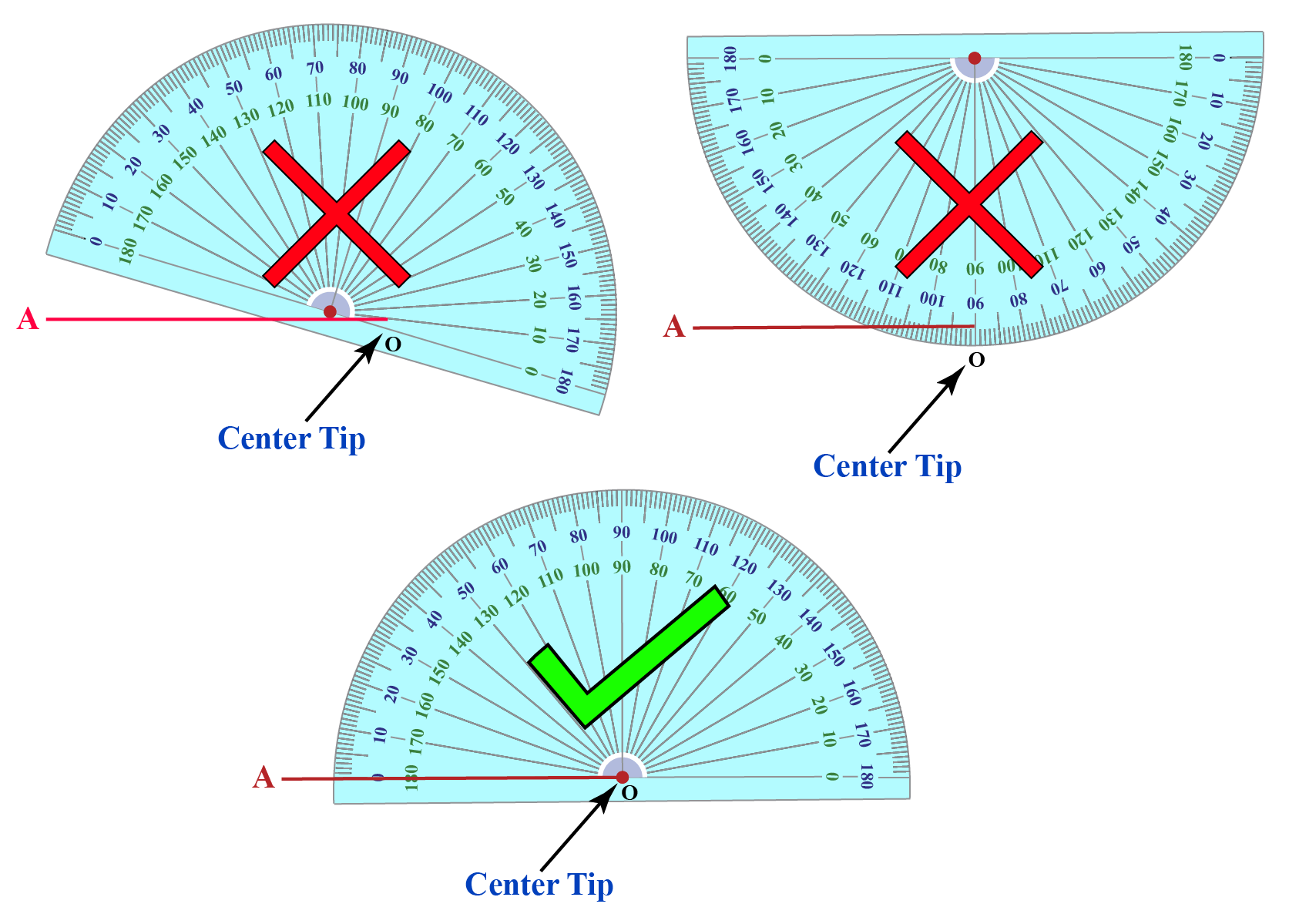Step 3: Start from 0 on the protractor in the clockwise direction and stop at 30. Mark point D.
If point A lies to the right of O, then start measuring anticlockwise and stop at 30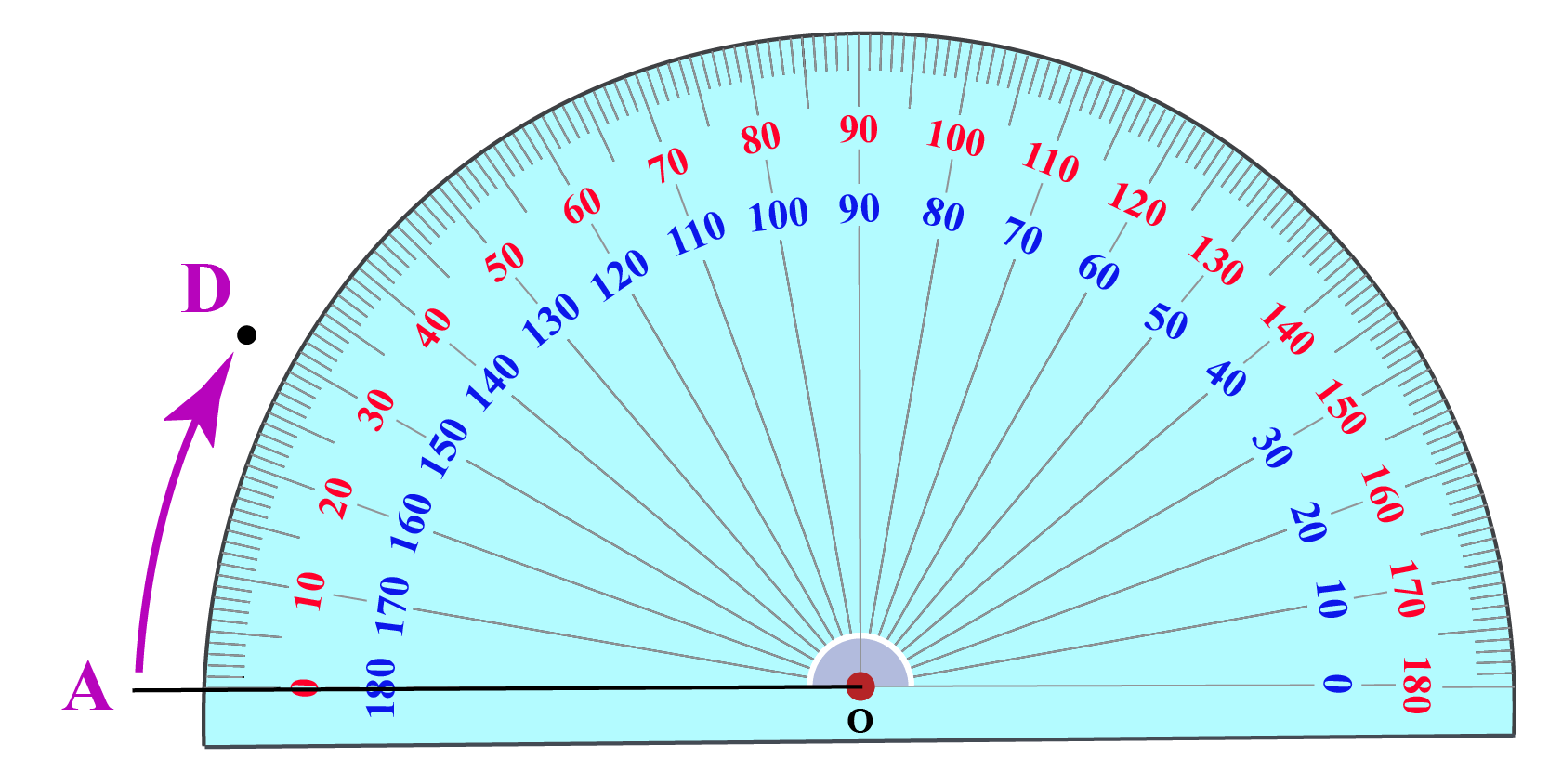Step 4: Join the point D with O. The $$\angle \text{AOC} = 30^{\circ}$$ is the required angle.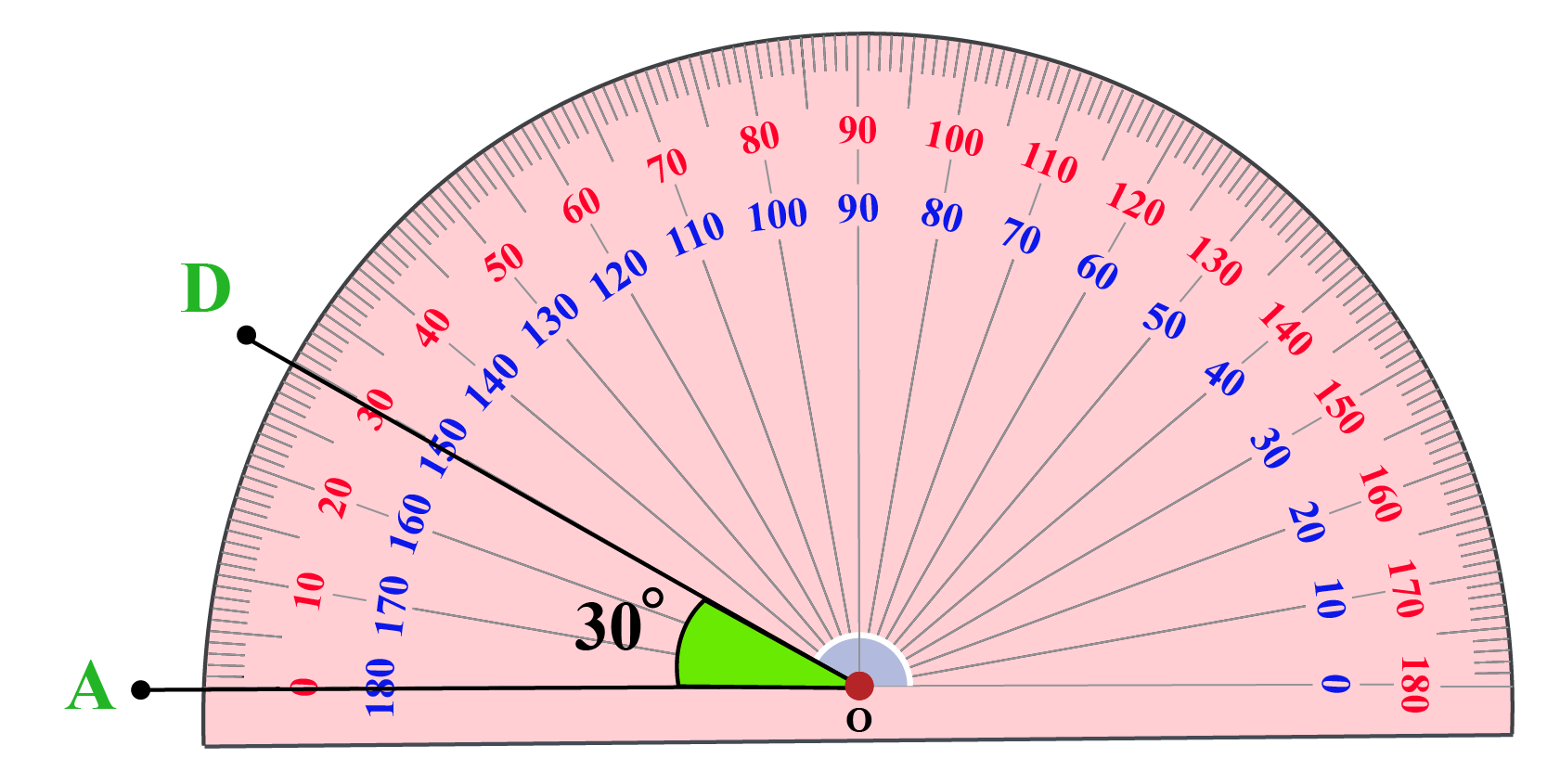Click on the Go button to understand the process of construction of a 30-degree angle using a protractor with the help of animation.

## What Are 30-Degree Angles in Real Life?

The 30-degree angle can be seen in many objects around us.

The hands of an analog clock at 1:00 PM forms an angle of 30 degrees.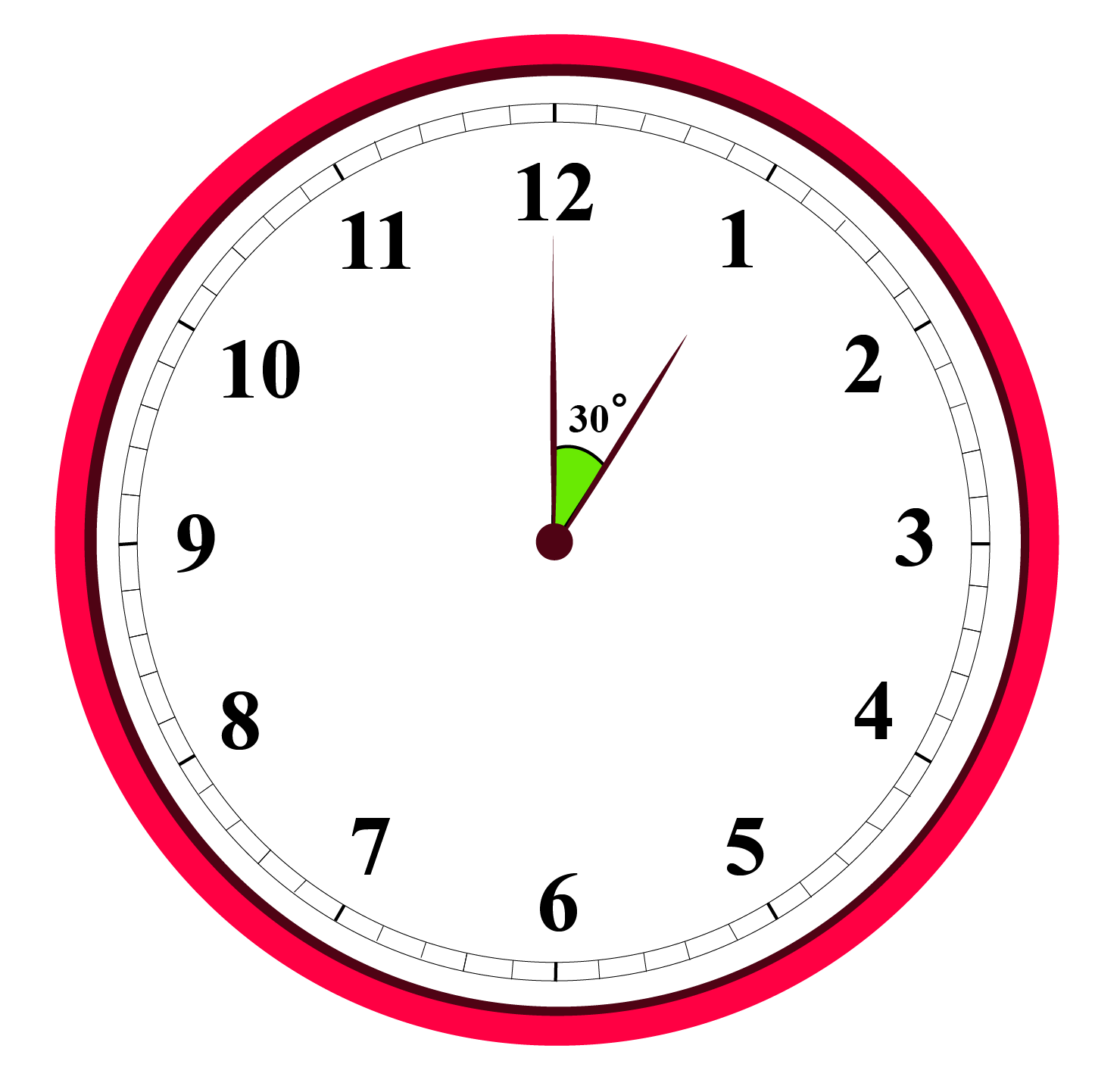If many friends come to your house and you need to cut the pizza into 12 slices, each slice of the pizza has a 30-degree angle.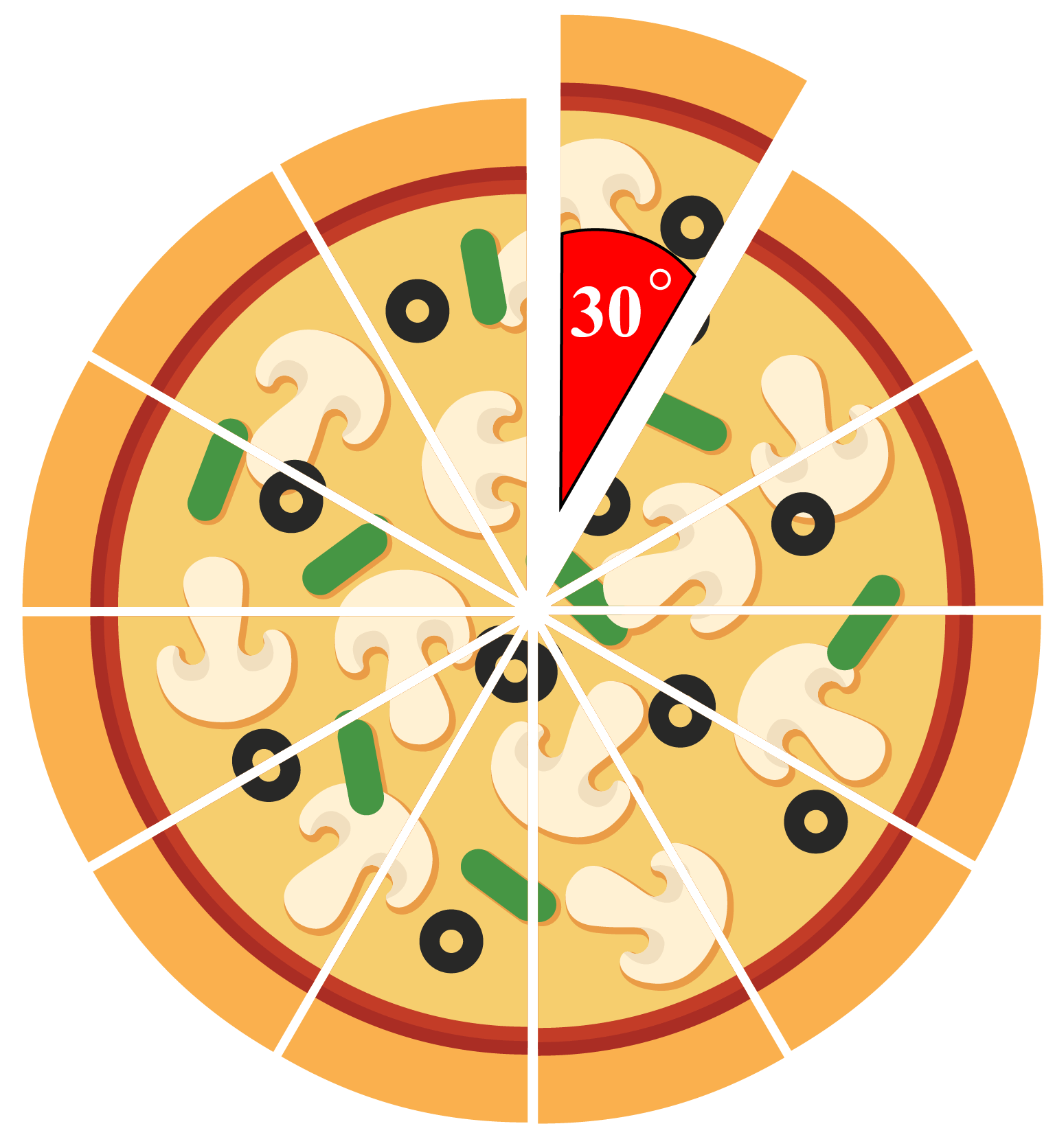Sometimes, the scissors we use to cut paper and cloth makes a 30-degree angle.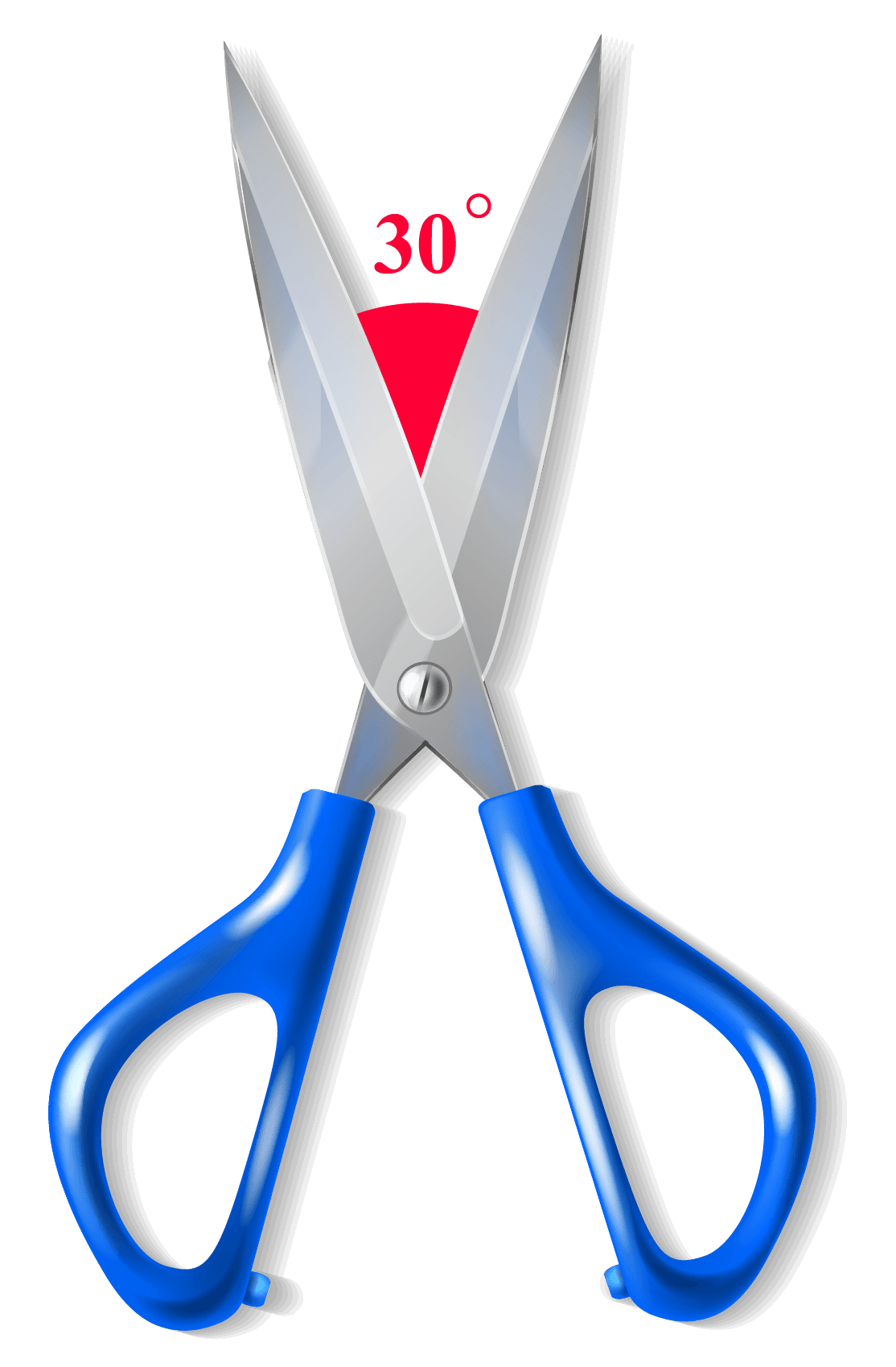Have you ever gone for a cycle ride with your friends and observed that the roads are diverging at some angles. Those angles could be 30-degree angles.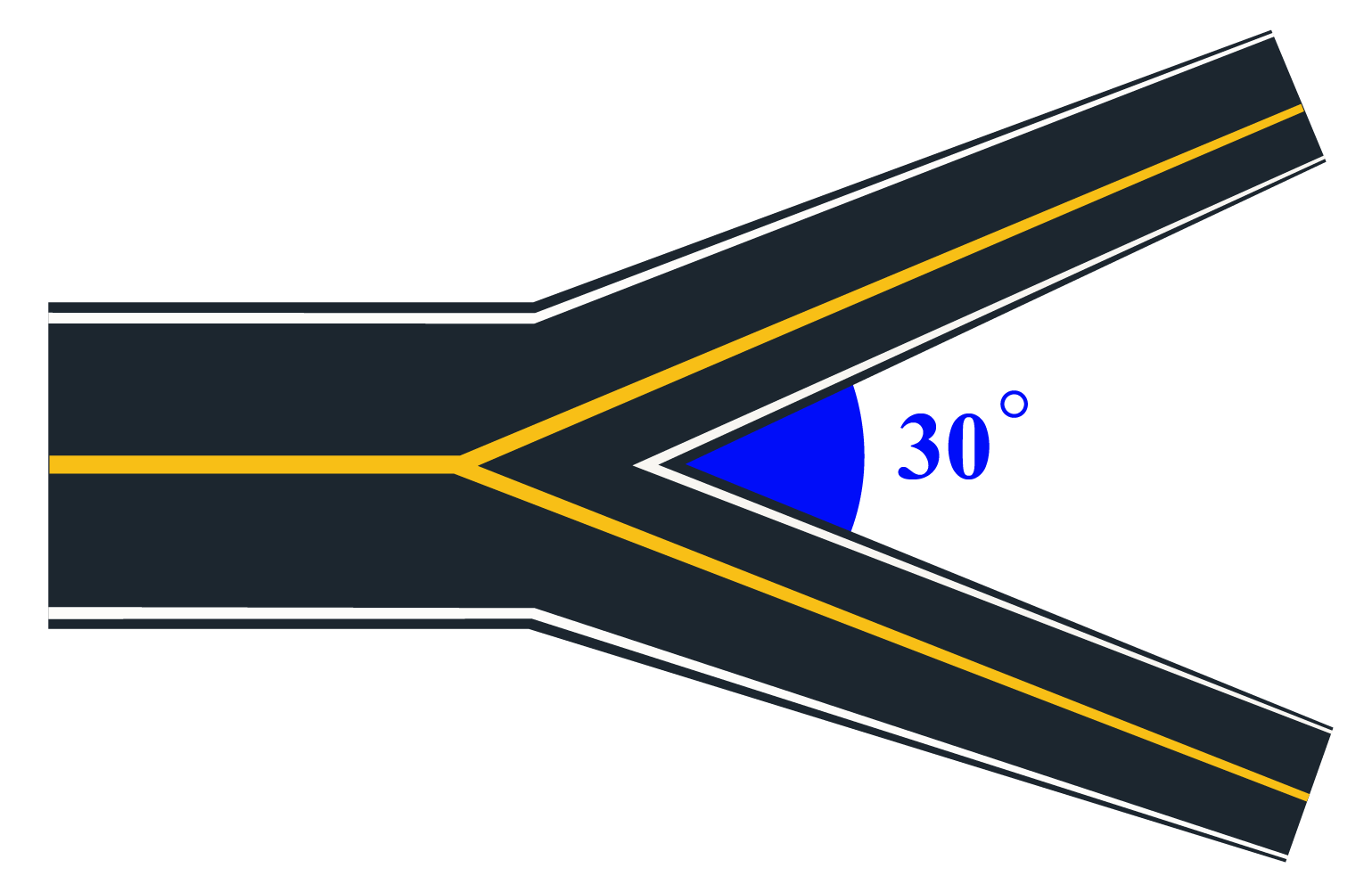Think Tank
1. How many 30-degree angles are there in a
a) Straight angle
b) Complete angle

## Solved Examples

 Example 1

In the $$\triangle \text{ABC}$$, use a protractor to measure $$\angle \text{CAB}$$.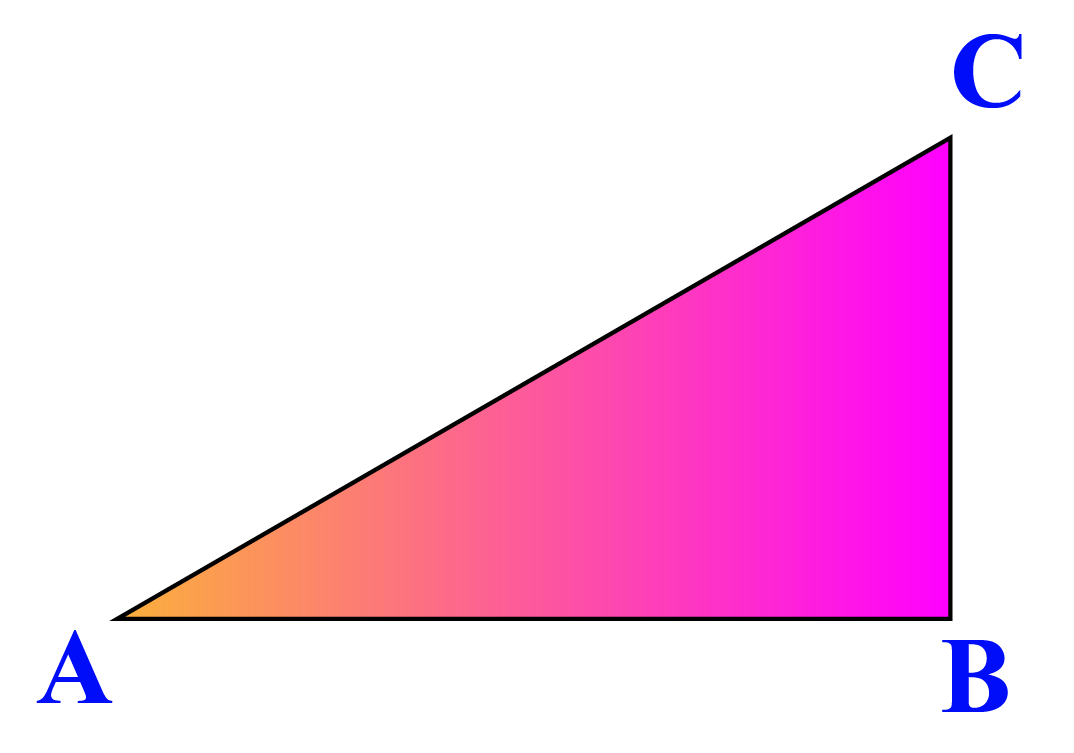Solution

Place the protractor's baseline along the line $$\text{AB}$$ and the center of the protractor at the vertex $$\text{A}$$.

Observe the reading in the protractor which overlaps with line $$\text{AC}$$.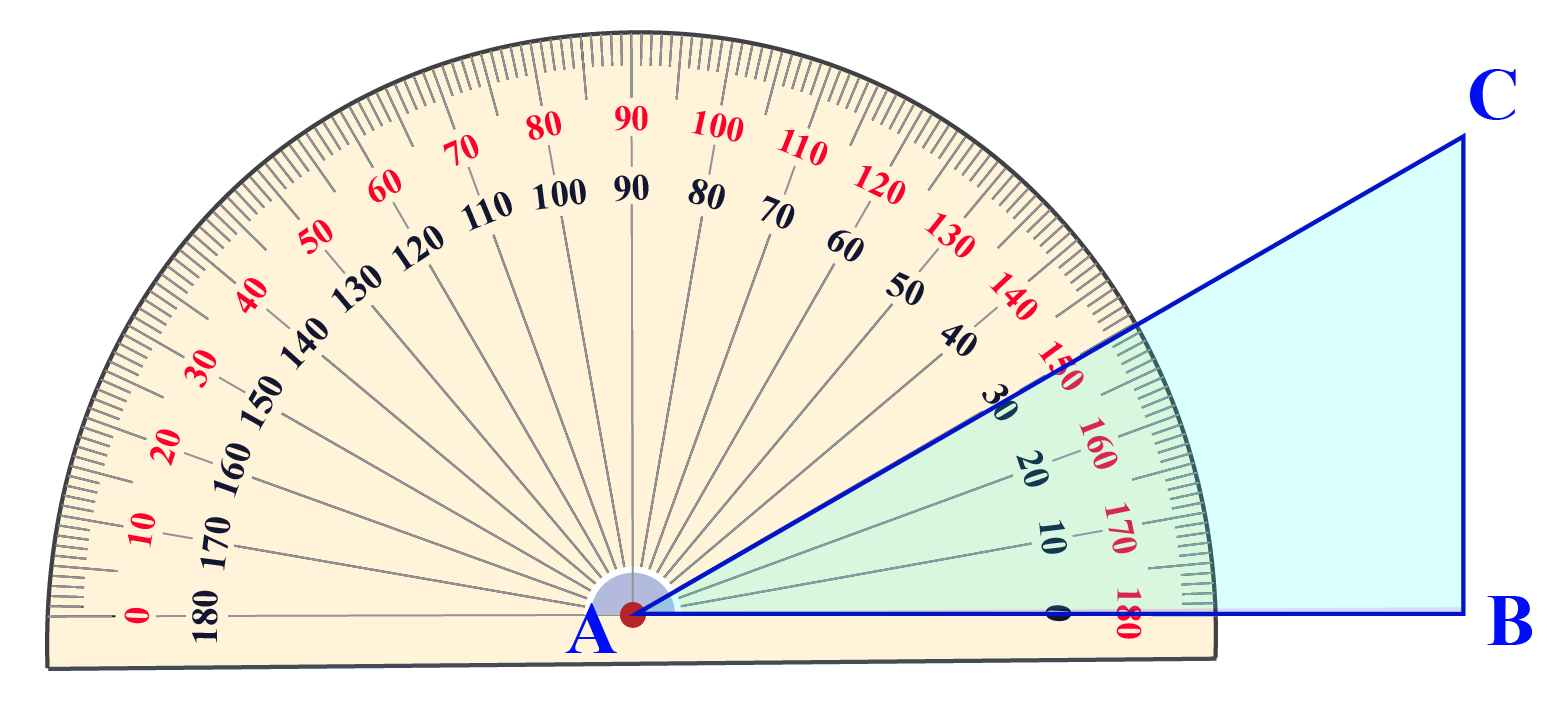$$\therefore \angle \text{CAB} = 30^\circ$$
 Example 2

Mathew ordered a large pizza at his home. Some time later, few of his friends came to his house. Mathew called the pizza shop and asked them to cut the pizza such that he would get 25% of the pizza and each of his friends would get a piece with a 30-degree angle. If each friend got one piece, how many friends came to Mathew's place?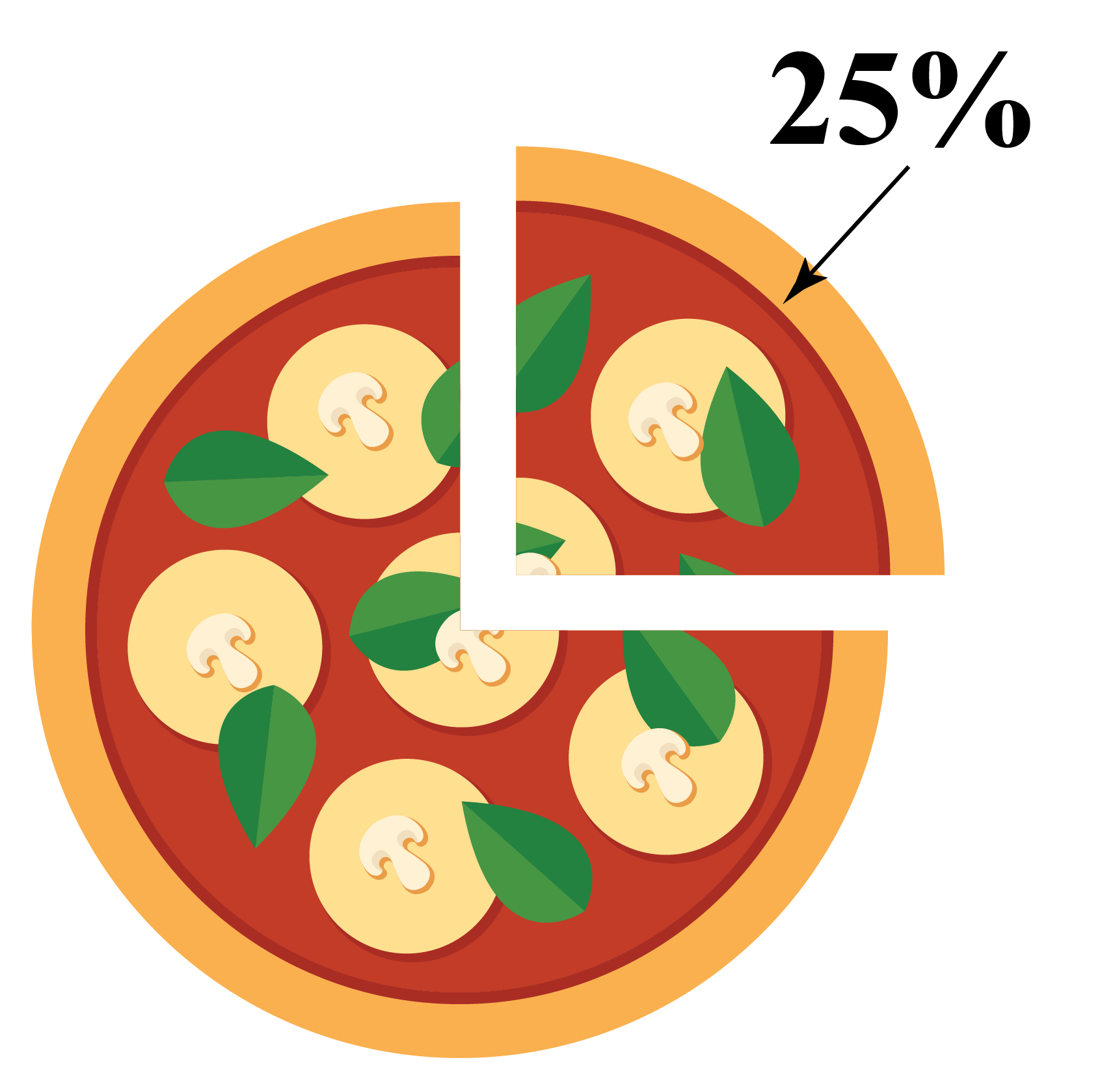Solution

The pizza is circular; hence the total angle of the pizza is $${\text{360}}^{\circ}$$.

Mathew is eating 25% of the pizza.

$= \text{25% of } {\text{360}}^{\circ} \\[0.2cm] = \dfrac{25}{100} \times {\text{360}}^{\circ} \\[0.2cm] = \dfrac{1}{4} \times {\text{360}}^{\circ} \\[0.2cm] = {\text{90}}^{\circ}$

Mathew is eating a piece with $${\text{90}}^{\circ}$$ angle.

The remaining pizza will be distributed among his friends equally.

${\text{360}}^{\circ} - {\text{90}}^{\circ} = {\text{270}}^{\circ}$

Now, Mathew's friends will eat $${\text{270}}^{\circ}$$ pizza.

Each pizza slice has an angle of 30 degrees.

Therefore, total number of friends that came at Mathew's house are

$= \dfrac{\text{Total angle of pizza remaining}}{\text{Angle of each slice}} \\[0.2cm] = \dfrac{\text{270}}{30} \\[0.2cm] = 9$

 $$\therefore \text{9 friends came at Mathew's house.}$$

More Important Topics
Numbers
Algebra
Geometry
Measurement
Money
Data
Trigonometry
Calculus